### Maximum Likelihood

The GLIMMIX procedure forms the log likelihoods of generalized linear models as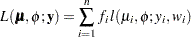whereis the log likelihood contribution of theth observation with weightandis the value of the frequency variable. For the determination ofand, see the WEIGHT and FREQ statements. The individual log likelihood contributions for the various distributions are as follows.

Beta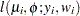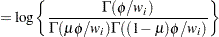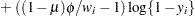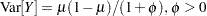. See Ferrari and Cribari-Neto (2004).

Binary.

Binomial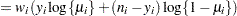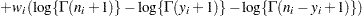whereand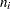are the events and trials in the events/trials syntax, and.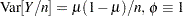.

Exponential.

Gamma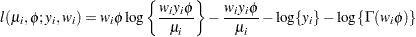.

Geometric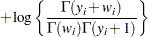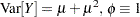.

Inverse Gaussian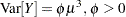.

"Lognormal"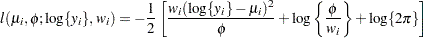.
If you specify DIST=LOGNORMAL with response variable Y, the GLIMMIX procedure assumes that. Note that the preceding density is not the density of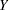.

Multinomial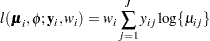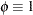.

Negative Binomial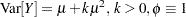.
For a given, the negative binomial distribution is a member of the exponential family. The parameteris related to the scale of the data, because it is part of the variance function. However, it cannot be factored from the variance, as is the case with theparameter in many other distributions. The parameteris designated as "Scale" in the "Parameter Estimates" table of the GLIMMIX procedure.

Normal (Gaussian)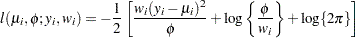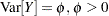.

Poisson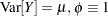.

Shifted T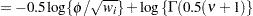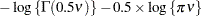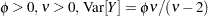.

Define the parameter vector for the generalized linear model as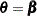, if, and asotherwise.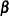denotes the fixed-effects parameters in the linear predictor. For the negative binomial distribution, the relevant parameter vector is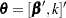. The gradient and Hessian of the negative log likelihood are then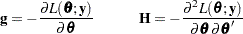The GLIMMIX procedure computes the gradient vector and Hessian matrix analytically, unless your programming statements involve functions whose derivatives are determined by finite differences. If the procedure is in scoring mode,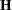is replaced by its expected value. PROC GLIMMIX is in scoring mode when the numberof SCORING=iterations has not been exceeded and the optimization technique uses second derivatives, or when the Hessian is computed at convergence and the EXPHESSIAN option is in effect. Note that the objective function is the negative log likelihood when the GLIMMIX procedure fits a GLM model. The procedure performs a minimization problem in this case.

In models for independent data with known distribution, parameter estimates are obtained by the method of maximum likelihood. No parameters are profiled from the optimization. The default optimization technique for GLMs is the Newton-Raphson algorithm, except for Gaussian models with identity link, which do not require iterative model fitting. In the case of a Gaussian model, the scale parameter is estimated by restricted maximum likelihood, because this estimate is unbiased. The results from the GLIMMIX procedure agree with those from the GLM and REG procedure for such models. You can obtain the maximum likelihood estimate of the scale parameter with the NOREML option in the PROC GLIMMIX statement. To change the optimization algorithm, use the TECHNIQUE= option in the NLOPTIONS statement.

Standard errors of the parameter estimates are obtained from the inverse of the (observed or expected) second derivative matrix.